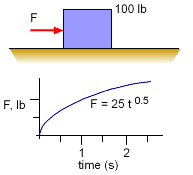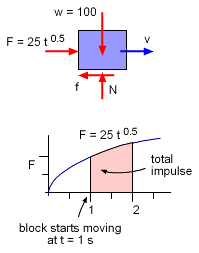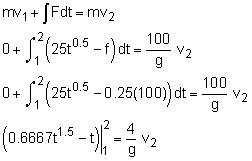Ch 4. Particle Momentum and Impulse Multimedia Engineering Dynamics Impulse & Momentum Consv. Linear Momentum Impact AngularMomentum MassFlow
 Chapter - Particle - 1. General Motion 2. Force & Accel. 3. Energy 4. Momentum - Rigid Body - 5. General Motion 6. Force & Accel. 7. Energy 8. Momentum 9. 3-D Motion 10. Vibrations Appendix Basic Math Units Basic Equations Sections Search eBooks Dynamics Fluids Math Mechanics Statics Thermodynamics Author(s): Kurt Gramoll ©Kurt GramollDYNAMICS - EXAMPLEBox Force Example A box that weighs 100 lb is pushed along a surface by a variable force, F = 25 t0.5 lb. The coefficient of friction between the box and the floor is 0.25 for both static and kinetic friction. What is the velocity of the box after 2 seconds? Assume the box starts from rest. SolutionImpulse Action on Box It is important first to note that t he block will not move until the force is large enough to overcome static friction. Thus, the exact time the box first moves needs to be determined. The block will move when the force is equal to friction force to move. Summing the forces in the x-direction, gives,      25 t0.5 - f = 0      μN = 25 t0.5       0.25 (100) = 25 t0.5      t = 1.0 s The motion of the box will depend on the linear impulse between 1 and 2 seconds.(1.8856 - 2) - (0.6667 - 1) = 0.1242 v2 v2 = 1.763 ft/s

Practice Homework and Test problems now available in the 'Eng Dynamics' mobile app
Includes over 400 problems with complete detailed solutions.
Available now at the Google Play Store and Apple App Store.